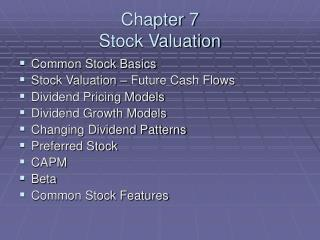DownloadDownload PresentationChapter 7 Stock Valuation

# Chapter 7 Stock Valuation

Télécharger la présentation## Chapter 7 Stock Valuation

- - - - - - - - - - - - - - - - - - - - - - - - - - - E N D - - - - - - - - - - - - - - - - - - - - - - - - - - -
##### Presentation Transcript

1. Chapter 7Stock Valuation • Common Stock Basics • Stock Valuation – Future Cash Flows • Dividend Pricing Models • Dividend Growth Models • Changing Dividend Patterns • Preferred Stock • CAPM • Beta • Common Stock Features

2. Common Stock Basics • Common Stock – Ownership of Company • Equity financing • Major financing source for companies • Voting Rights • Pick the management team of the company • Determine charter and changes to charter • Receive Dividends • Payment of “profits” • Principal not repaid until shareholder sells stock

3. Stock Valuation • Just like a bond…the value is all future cash flows received • Final payment (when stock is sold) is the same as principal repayment • Dividends are the same as coupons

4. Dividend Pricing Models • Four Models • Two assumptions • Growth, constant or no-growth dividend or dividend growing at a constant rate • Horizon, finite or infinite • Constant Dividend and Infinite Horizon • Priced as a perpetuity • Price = Dividend / r • r is the desired rate of return on investment

5. Dividend Pricing Models • Constant Dividend with Finite Horizon • Assumption on final payment • Dividends Only – Only annuity stream formula • Dividends and Final Sale at end of horizon – Annuity Stream and Lump Sum formula • TVM equations • Finding Bond Selling Price • New owner buys remaining dividend stream • Perpetuity for new owner?

6. Dividend Growth Models • Common practice to raise dividends each year • Example, Coca-Cola • Dividend changes range • \$0.04 to \$0.08 • 5.88% to 14.71% • Not constant…but… • Smooth out pattern for constant percentage change each year • Constant percentage change allows for a simplified pricing model

7. Dividend Growth Models • First Constant Growth Model – Infinite Horizon • Need g, the dividend growth rate • Need r, the require rate of return • Both stated on annual basis

8. Dividend Growth Models • Finding g, • Averaging annual dividend changes • g = (Last Dividend / First Dividend)1/n • n is the number of dividend changes • Example of Johnson and Johnson, g = 13.8% • Select r, example J&J, r = 15%

9. Dividend Growth Models • Adjusted for Finite Horizon • Dividend stream for a fixed period of time but still has constant growth, g>0 • Shareholder only gets a portion of infinite dividend stream • Add portion adjustment to model

10. Changing Dividend Patterns • What happens when g is not constant or the dividends are not constant? • Look for patterns inside the overall dividend stream • Series of dividends with constant dividends • Series of dividends with constant growth • Apply models within the series finding PV of the series • Add up all present value pieces for price

11. Preferred Stock • Preferred stock has stated annual dividend • Constant dividend each period • Dividend = dividend rate x par value • Preferred stock has no maturity date • Infinite horizon • No payment of par value • Fits constant dividend model with infinite horizon

12. CAPM • Capital Asset Pricing Model – CAPM • Future cash flows (dividends) • Difficult to predict • May not follow nice patterns • More encompassing model • CAPM – Expected return is directly related to risk (Chapter 5, Risk and Return) • Model works with returns

13. CAPM • Assumptions • #1 – There is a reward for waiting • #2 – The greater the risk the greater the expected reward • #3 – There is a constant tradeoff between risk and reward • E(return) = risk-free rate + slope (level of risk) • Trick is to find the level of risk for an investment

14. Beta • Beta – A measure of risk • The systematic risk of an individual asset in a well-diversified portfolio • Correlation between an asset’s returns and the market in general • Estimated with OLS regression • Beta’s • Less than 1, 1 or greater than 1 • Less risk than market, same risk as market, greater risk than the market

15. Beta • Using Beta • Security Market Line • All firms plot on SML (ex-ante) • Firms above the line are under priced • Firms below the line are over priced • Security Market Line estimates expected returns

16. Common Stock Features • Claim on Assets and Cash Flows (Residual) • No Maturity Date • Vote (Voice in Management) • Tax Issues – Dividends Taxable • Other Issues • Authorized, Outstanding, Treasury Shares • Pre-emptive Rights

17. Problems • Problem 1 – Constant Dividend, Infinite Horizon • Problem 2 – Constant Dividend, Infinite Horizon • Problem 3 – Constant Dividend, Infinite Horizon • Problem 5 – Constant Growth, Infinite Horizon • Problem 7 – Constant Growth, Finite Horizon • Problem 11 – Preferred Stock • Problem 15 – Security Market Line • Problem 17 – Expected Returns